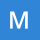Home Communities
IT Knowledge
Inspiration
Languages
EN

# JavaScript - One-time Pad encryption with XOR

9 points
Created by:Majid-Hajibaba
462

In this short article, we would like to show a simple JavaScript implementation of the most save encrytion that was invented by humans - One-time Pad encryption with XOR.

Used XOR formula:

``````Encryption:

00110100  input byte
XOR  11000111  secret key
--------
11110011  encrypted byte

Decription:

11110010  encrypted byte
XOR  11000111  secret key
--------
11110011  decrypted byte == input byte``````

Quick solution:

1. single byte encryption

``````// ONLINE-RUNNER:browser;

const inputByte = 97;
const secretKey = 238; // random byte used as secret key

const encryptedByte = inputByte ^ secretKey;     // Encryption
const decryptedByte = encryptedByte ^ secretKey; // Decription

console.log(inputByte);      // 97
console.log(encryptedByte);  // 143
console.log(decryptedByte);  // 97``````

2. multiple bytes encryption

``````// ONLINE-RUNNER:browser;

const inputBytes = [97, 98, 99];    // what is 'abc' when we use UTF-8 bytes
const secretKeys = [238, 203, 230]; // 3 random bytes used as secret keys

// Encryption:

const encryptedBytes = Array(inputBytes.length);
for (let i = 0; i < inputBytes.length; ++i) {
encryptedBytes[i] = inputBytes[i] ^ secretKeys[i];
}

// Decription:

const decryptedBytes = Array(encryptedBytes.length);
for (let i = 0; i < encryptedBytes.length; ++i) {
decryptedBytes[i] = encryptedBytes[i] ^ secretKeys[i];
}

console.log(inputBytes);      // [97, 98, 99]
console.log(encryptedBytes);  // [143, 169, 133]
console.log(decryptedBytes);  // [97, 98, 99]``````

## Algorithm description

The algorithm is as strong as perfectly random numbers have been used as secret keys.

The main concept of the algorithm is to:

1. use XOR formula on the data units to encode and decode,

Hint: check Wikipedia reference to see other than XOR encryption formula.

2. store on sender and recipient devices, secret keys composed of perfect random numbers
(the numbers from computer algorithms may be predicatable, so use nature based approaches),
3. never share the random numbers with others than the sender and the recipient,
4. use always only part of secret keys to encrypt or decrypt some sent message (one byte encoded by one secret key),
5. never use 2 times the same secret keys.

## Universal implementation

This implementation provides cipher that stores information about lastly used keys, preventing against bereaking rule 5 (never use 2 times the same secret key).

``````// ONLINE-RUNNER:browser;

// One-time Pad encryption with XOR

const Cipher = (secretKeys) => {
const createScope = () => {
let secretOffset = 0;
const execute = (inputBytes) => {
if (secretOffset + inputBytes.length > secretKeys.length) {
throw new Error('There is not enought secret keys to encode / decode bytes.');
}
const outputBytes = Array(inputBytes.length);
for (let i = 0; i < inputBytes.length; i += 1, secretOffset += 1) {
outputBytes[i] = secretKeys[secretOffset] ^ inputBytes[i];
}
return outputBytes;
};
return execute;
};
return {
encrypt: createScope(),
decrypt: createScope()  // to decrypt we use same formula like in the encryption case
};
};

// Usage example:

// common - the information that know both (sender and recipient)

// in our case we use random bytes - be sure that the values are from 0 to 255
const secretKeys = [238, 203, 230, 91, 223, 161, 59, 10, 105, 200, 45, 145, 64, 145, 210]; // random numbers sequence used as secret key

// sender

const senderCipher = Cipher(secretKeys);

const encryptedBytes1 = senderCipher.encrypt([97, 98, 99]); // what is 'abc' as UTF-8 bytes
const encryptedBytes2 = senderCipher.encrypt([49, 50, 51]); // what is '123' as UTF-8 bytes
const encryptedBytes3 = senderCipher.encrypt([97, 98, 99]); // what is 'abc' as UTF-8 bytes

console.log(`Encrypted 1: \${encryptedBytes1}`);  // Encrypted 1: [143, 169, 133]
console.log(`Encrypted 2: \${encryptedBytes2}`);  // Encrypted 2: [106, 237, 146]
console.log(`Encrypted 3: \${encryptedBytes3}`);  // Encrypted 3: [90, 104, 10]

// recipient

const recipientCipher = Cipher(secretKeys);

const decryptedBytes1 = recipientCipher.decrypt(encryptedBytes1);
const decryptedBytes2 = recipientCipher.decrypt(encryptedBytes2);
const decryptedBytes3 = recipientCipher.decrypt(encryptedBytes3);

console.log(`Decrypted 1: \${decryptedBytes1}`);  // Decrypted 1: [97, 98, 99]
console.log(`Decrypted 2: \${decryptedBytes2}`);  // Decrypted 2: [49, 50, 51]
console.log(`Decrypted 3: \${decryptedBytes3}`);  // Decrypted 3: [97, 98, 99]``````

Conclusions:

• `[97,98,99]` encrypted 2 times returned 2 different results: `[143,169,133]` and `[90,104,10]` - it is provided by once time secret key usage composed of random numbers.

## Alternative implementation

``````const maskBytes = (offset, key, bytes) => {
const result = Array(bytes.length);
for (let i = 0; i < bytes.length; ++i) {
result[i] = key[offset + i] ^ bytes[i];
}
return result;
};

const Cipher = (secret) => {
let offset = 0;
const execute = (bytes) => {
if (offset + bytes.length > secretSequence.length) {
throw new Error('There is not enought numbers in the secret sequence to encode / decode bytes.');
}
const result = maskBytes(offset, secret, bytes);
offset += result.length;
return result;
};
return {
encrypt: execute,
decrypt: execute
};
};``````